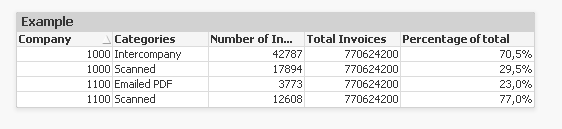QlikView Extensions

Discussion Board for collaboration on QlikView Extensions.

Highlighted
New Contributor III

Total in an expression

Hi, QV

I have one problem that I don't know he solution.

I am seeing if you can figure it out.

I have this dataset. I have information of number of invoices for every category and company.

 Company Categories No. of invoices 1000 Intercompany 42787 1000 Scanned 17894 1100 Emailed PDF 3773 1100 Scanned 12608

I want to know e.g.

How many invoices in percentage are scanned for company 1000 of total invoices for company 1000.

The answer I am looking for is:

Scanned Invoices for company 1000/ Total Invoices for company 1000 = 17894/60681 = 29,4%

I tried to use this formula: =Sum([Number of invoices])/Sum(Total [Number of invoices])

But it does not give me the right answer because in the formula: Sum(Total [Number of invoices]

I get all the invoices.

Tags (2)
1 Solution

Accepted SolutionsMVP

Re: Total in an expression

Use below expression

=Sum([Number of invoices])/Sum(Total <Company>[Number of invoices] )

4 RepliesMVP

Re: Total in an expression

Try this:

=Sum([Number of invoices] & Company)/Sum(Total <Company> [Number of invoices] & Company)MVP

Re: Total in an expression

Use below expression

=Sum([Number of invoices])/Sum(Total <Company>[Number of invoices] )MVP

Re: Total in an expression

You need to specify the company dimension in your TOTAL expression

Sum([Number of invoices])/Sum(Total <Company> [Number of invoices])

Use this in a chart with Company as a dimensionMVP

Re: Total in an expression

replace

Sum([Number of invoices])/Sum(Total [Number of invoices])

with

Sum([Number of invoices])/Sum(Total  <Company> [Number of invoices])

because you want the total at the Company level